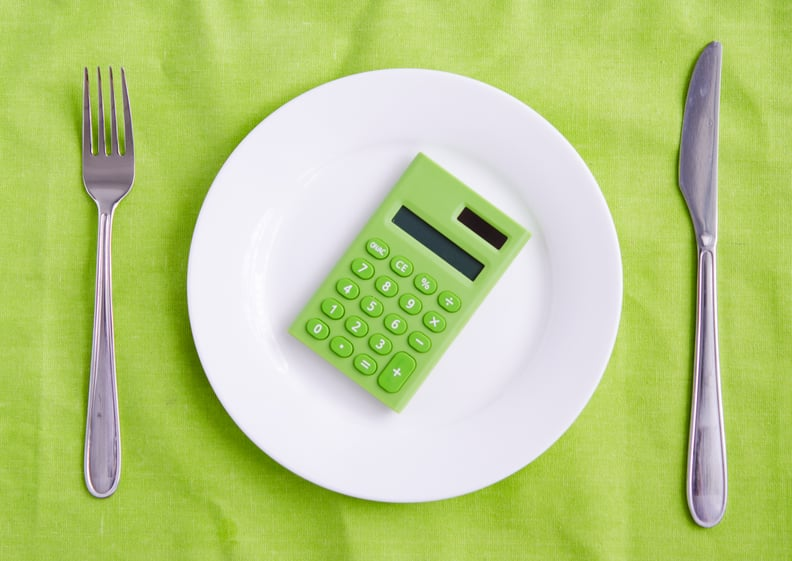# The Key to Weight Loss Is Calorie Deficit — Here's How to Calculate YoursAlthough there isn't a definitive, magical formula for weight loss, setting a calorie deficit is probably the closest thing. A calorie deficit occurs when you expend more calories than you take in. While the concept seems simple, finding your personal calorie deficit can take some trial and error. To help us understand this concept further and how it can help with weight loss, POPSUGAR spoke with registered dietitian nutritionist, Zara Awan. Ahead, find out what a calorie deficit is, how to find yours, and a formula to help you calculate it.

## What Is a Calorie Deficit?

"If you're in a calorie deficit, it means you took in less calories than your body needed to maintain its current weight," said Awan. "For most people being in a calorie deficit means you lose weight because when you are in one, your body will burn stored calories for energy," she continued. While a calorie deficit is helpful for weight loss, know that calories aren't the only contributing factor to how much weight you lose over time. "[T]here's a lot of factors that affect your weight loss, like having sex, stress, your sleeping patterns, your physical activity. So all of that also plays a huge role when it comes to weight loss."

## Calorie Deficit Calculator: Mifflin-St Jeor Equation

In order to find your calorie deficit you first need to find your basal metabolic rate (BMR). Your BMR is "how many calories you burn at rest," Awan explained. "Now, if you want to figure it out on your own, there's definitely multiple equations that you can use to try to find out what your basal metabolic rate is. The one that I use the most in my practice, it would be the Mifflin-St Jeor." According to Awan, here's how you find your basal metabolic rate (BMR) using the Mifflin-St Jeor equation.

1. Take your weight in kilograms, and multiply it by 10, leave that number alone
2. Take your height, in centimeters, and multiply by 6.25, leave that number alone
3. Take your age and multiply by five, leave that number alone
4. Take the number from step one and add it to the number from step two
5. Take the number from step four and subtract it from the number in step three
6. Take the number from step five and subtract it by 161
7. The number from step six is your BMR

Note: Awan made it clear that this will give you a solid estimate but the most accurate measurement or your BMR will come from equipment used by a doctor or dietitian during a visit.

If that felt like a lot of calculating, here's an example. Let's say you are 24 years old, weigh 300 pounds, and are five-foot-six-inches tall (136.1 kg and 167.6 cm); your equation would look like this:

1. 10 x 136.1 = 1,361
2. 6.25 x 167.6 = 1,047.5
3. 5 x 24 = 120
4. 1,361 + 1,047.5 = 2,408.5
5. 2,408.5 - 120 = 2,288.5
6. 2,288.5 - 161 = 2,127.5
7. Your BMR would be 2,127.5

Once you have your BMR you can subtract 250-500 calories to determine your calorie deficit as Awan said "each provider has their own numbers of what they think you need to reduce for your daily calorie range." So try out one deficit and adjust accordingly to suit your personal weight loss goals. For example Awan mentioned that, "there's 3,500 calories in one pound. If you want to lose one pound a week, you would have to be at a deficit of 500 calories per day." Using the example above that would be BMR - 500 = calorie deficit or 2,127.5 - 500 = 1,627.5.

Remember: There are many factors that go into one's ability to lose weight so don't beat yourself up if finding the calorie deficit (BMR minus 250 to 500) that works for your body takes more than a few tries.